# Lakhmir Singh Solutions Class 9 Physics Chapter 5 Sound

Sound is defined as the mechanical energy which is produced due to the vibrations of different objects. When the particles move back and forth from their central position there is a production of vibrations like in case of a guitar. When the strings of a guitar are struck, there is a production of sound. When these sound waves travel from one medium to another, it is known as the propagation of sound. For these vibrations to travel, a medium is required.

The medium could be solid, liquid, or gas. It is important to understand that sound waves cannot travel in a vacuum as there are no air particles to propagate the sound waves. Following are the characteristics of sound waves:

• Amplitude
• Wavelength
• Frequency
• Time period
• Velocity

The propagation of waves is based on the direction of propagation and there are two categories of waves:

• Longitudinal waves: When the waves have particles that vibrate along the direction of propagation of the waves. Sound waves are longitudinal waves.
• Transverse waves: When the waves have particles that vibrate perpendicular to the direction of propagation of the waves. Waves produced in a stretched string is an example of transverse waves.

Compression and rarefactions are the parts of the waves. When the particles of the medium are close to each other and this results in the formation of high pressure and density region, it is known as compression. This is represented by the upper portion of the curve which is known as the crest. When the particles of the medium are farther apart and this results in the formation of low pressure and density region, it is known as rarefaction. This is represented by the lower portion of the curve which is known as the trough.

## Download PDF Of Lakhmir Singh Physics Class 9 Solutions For Chapter 5 Sound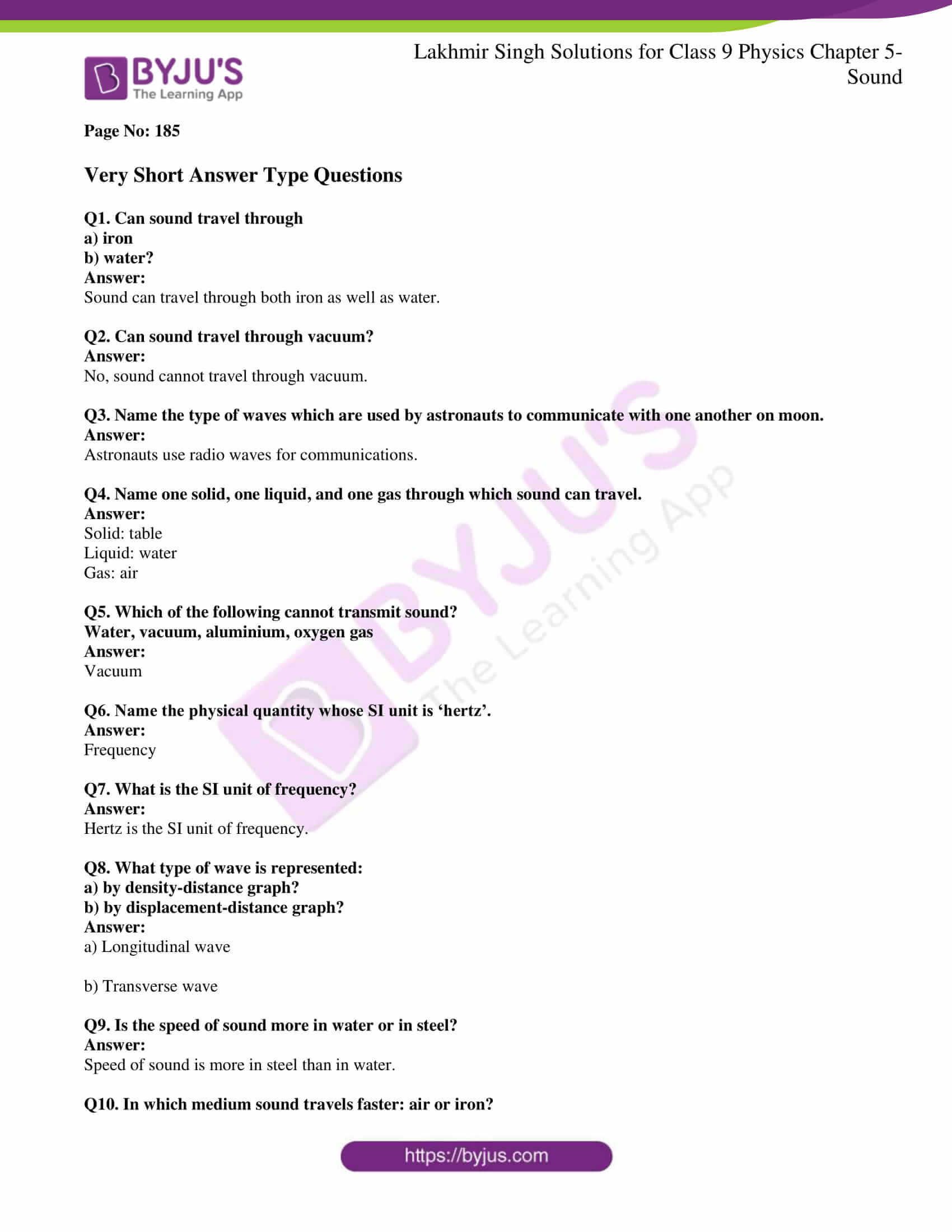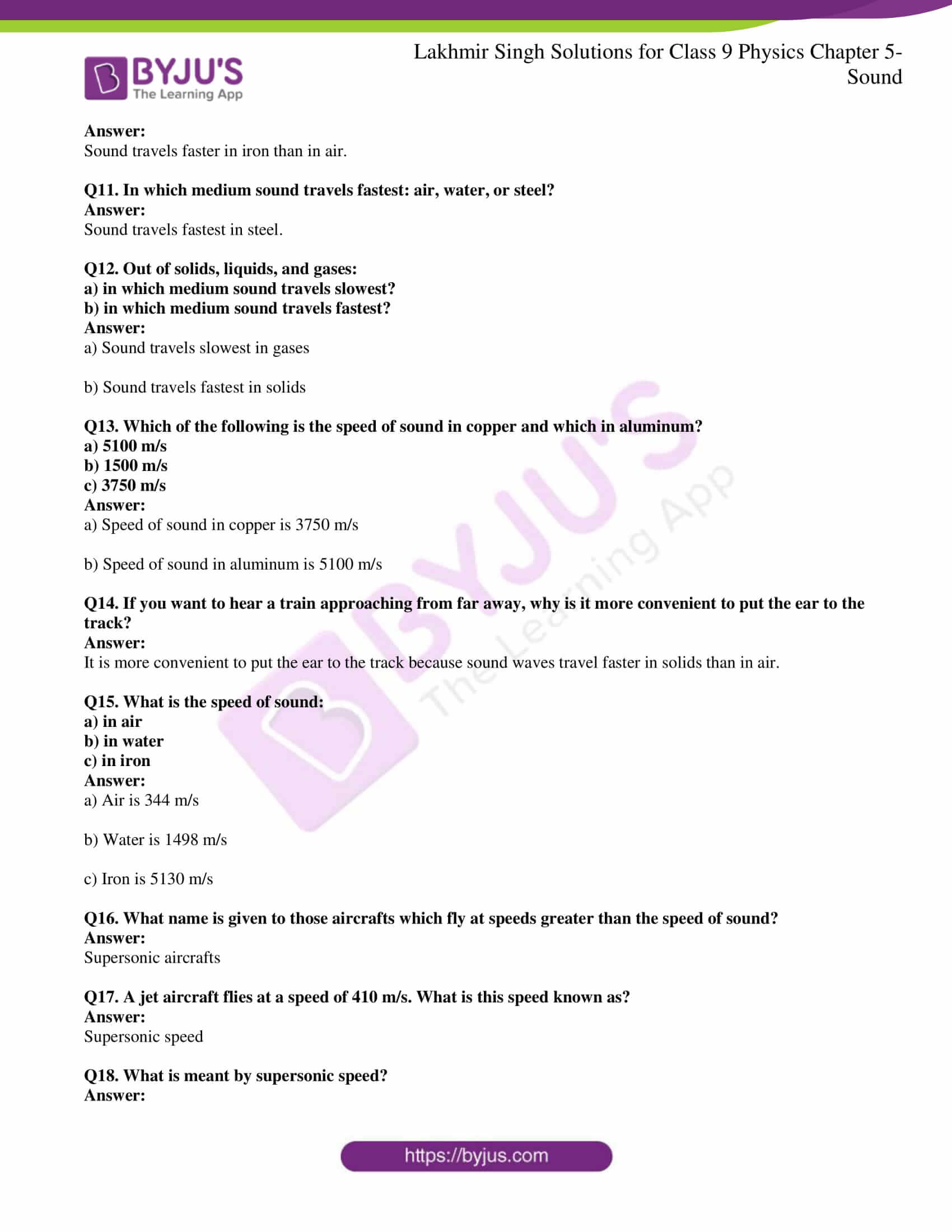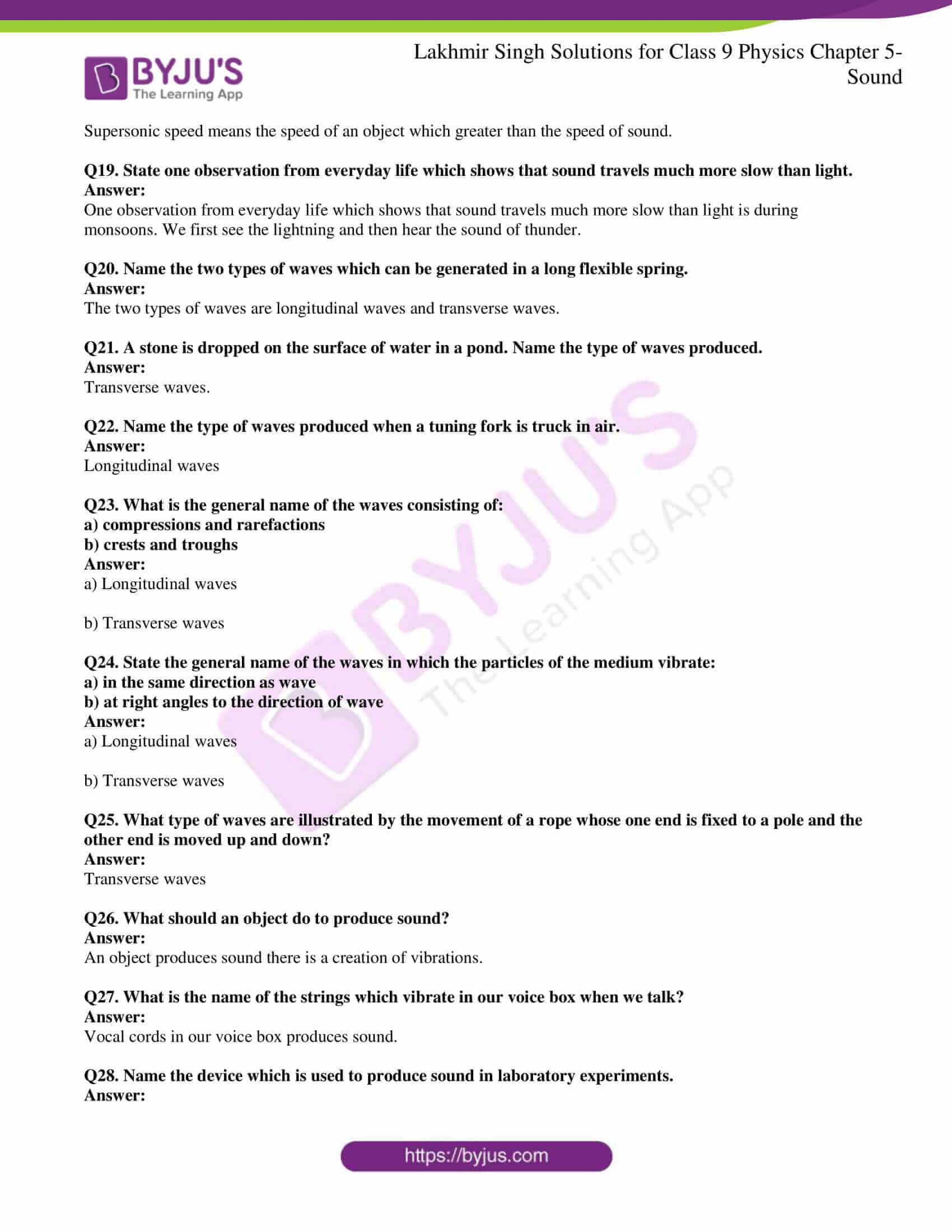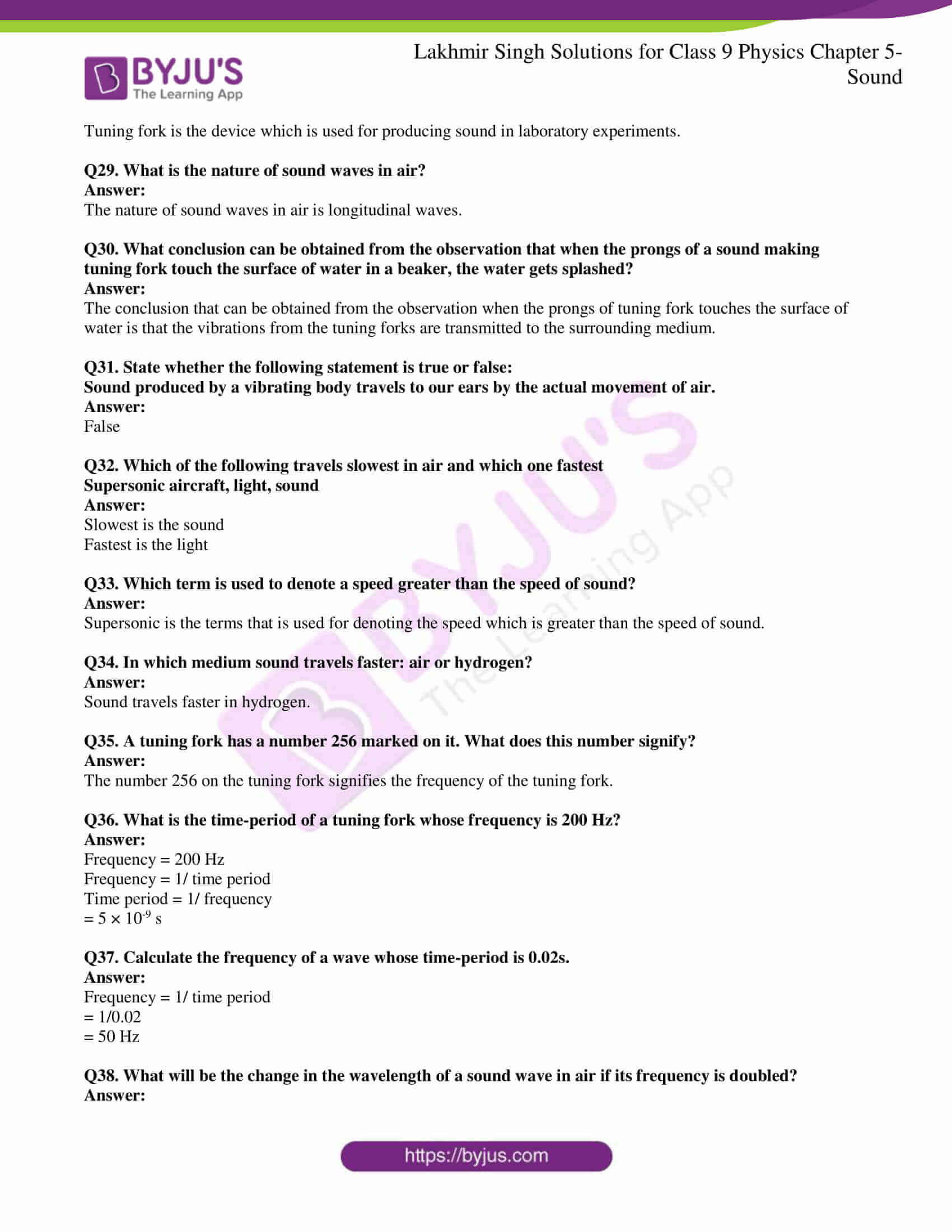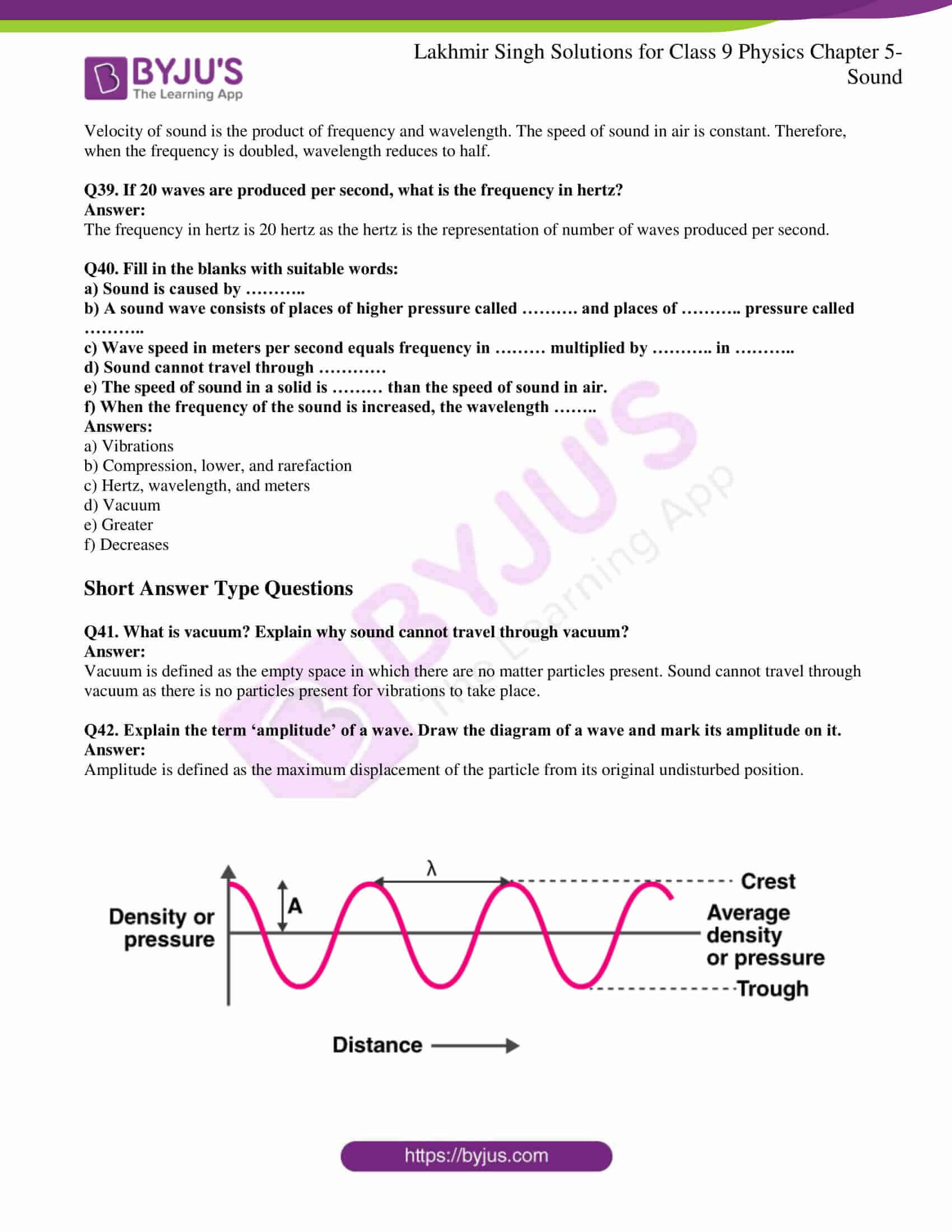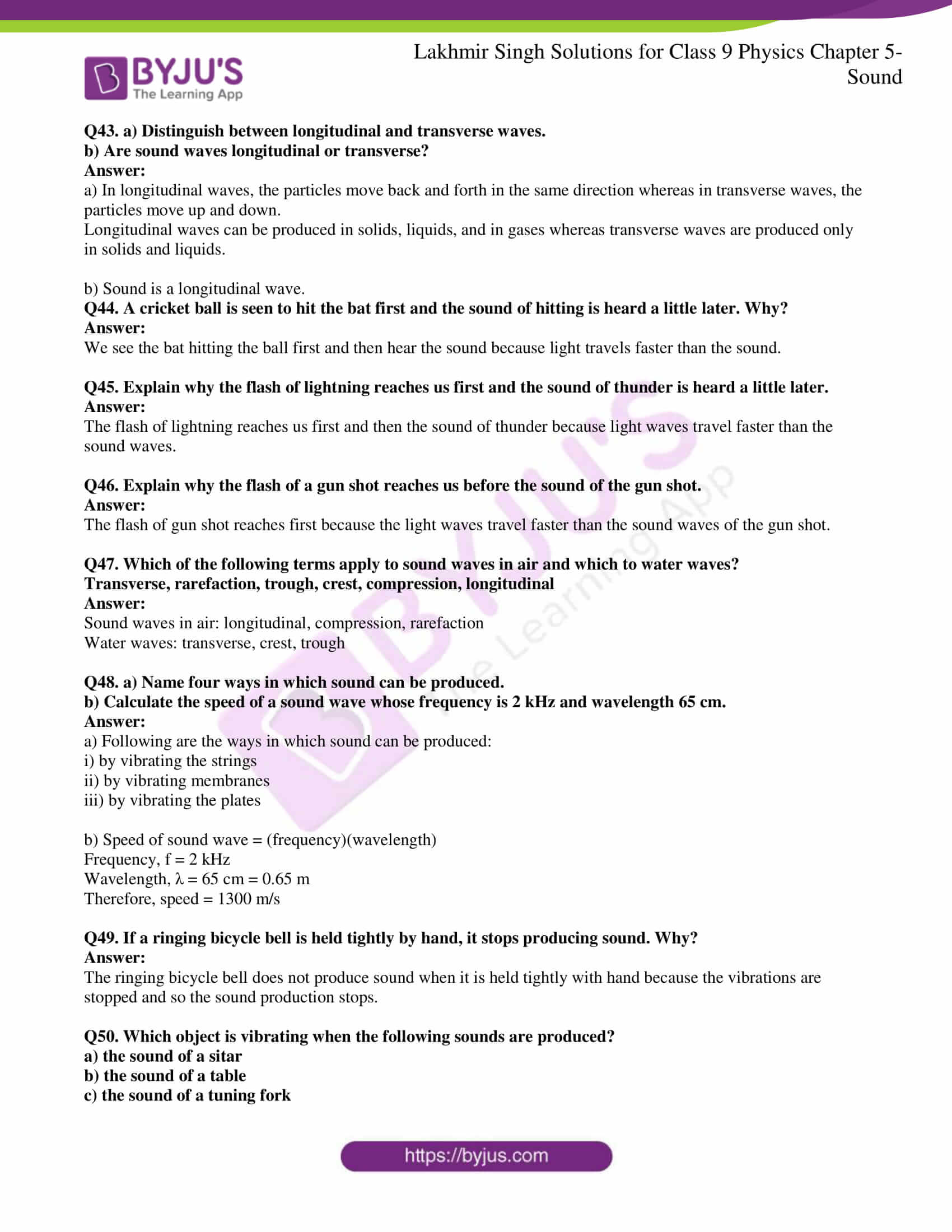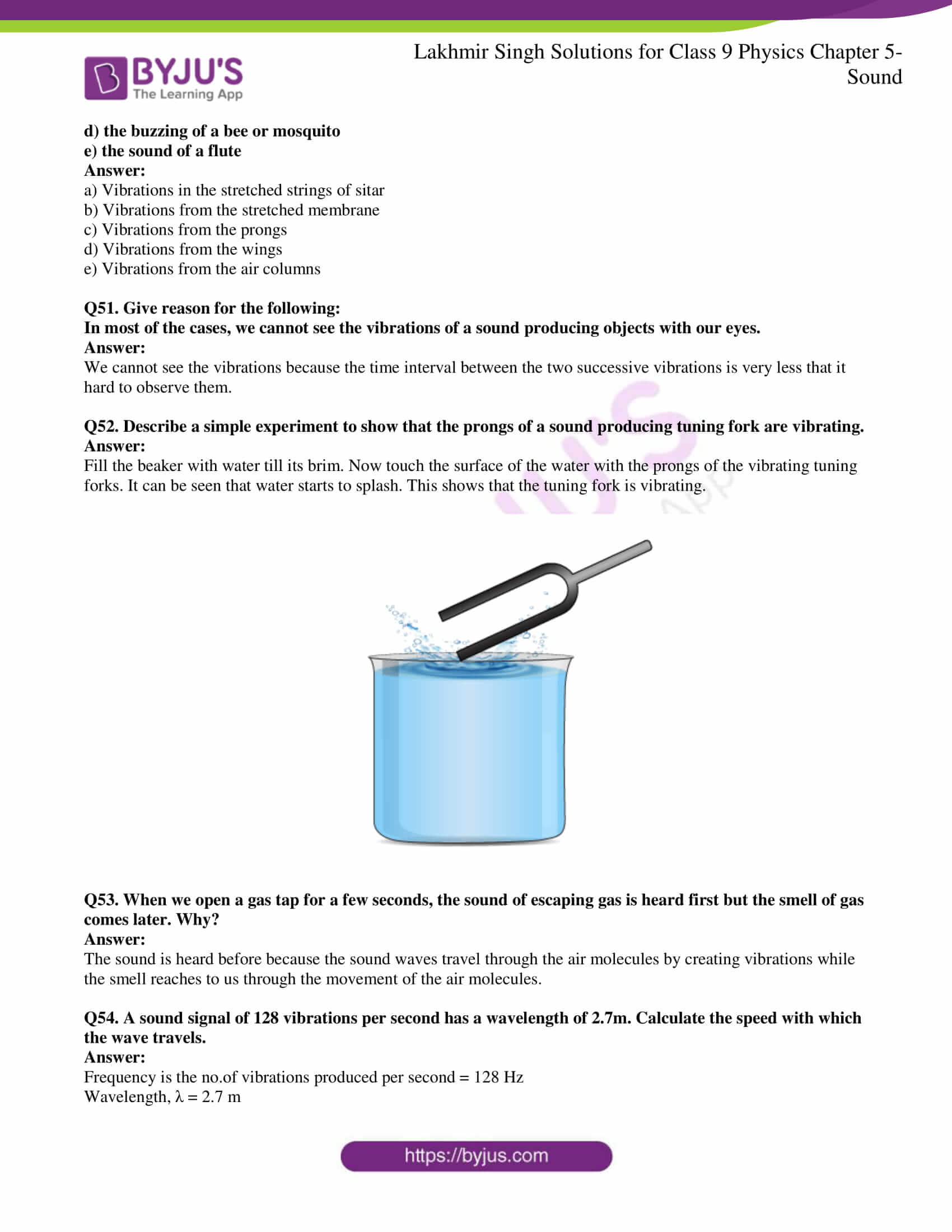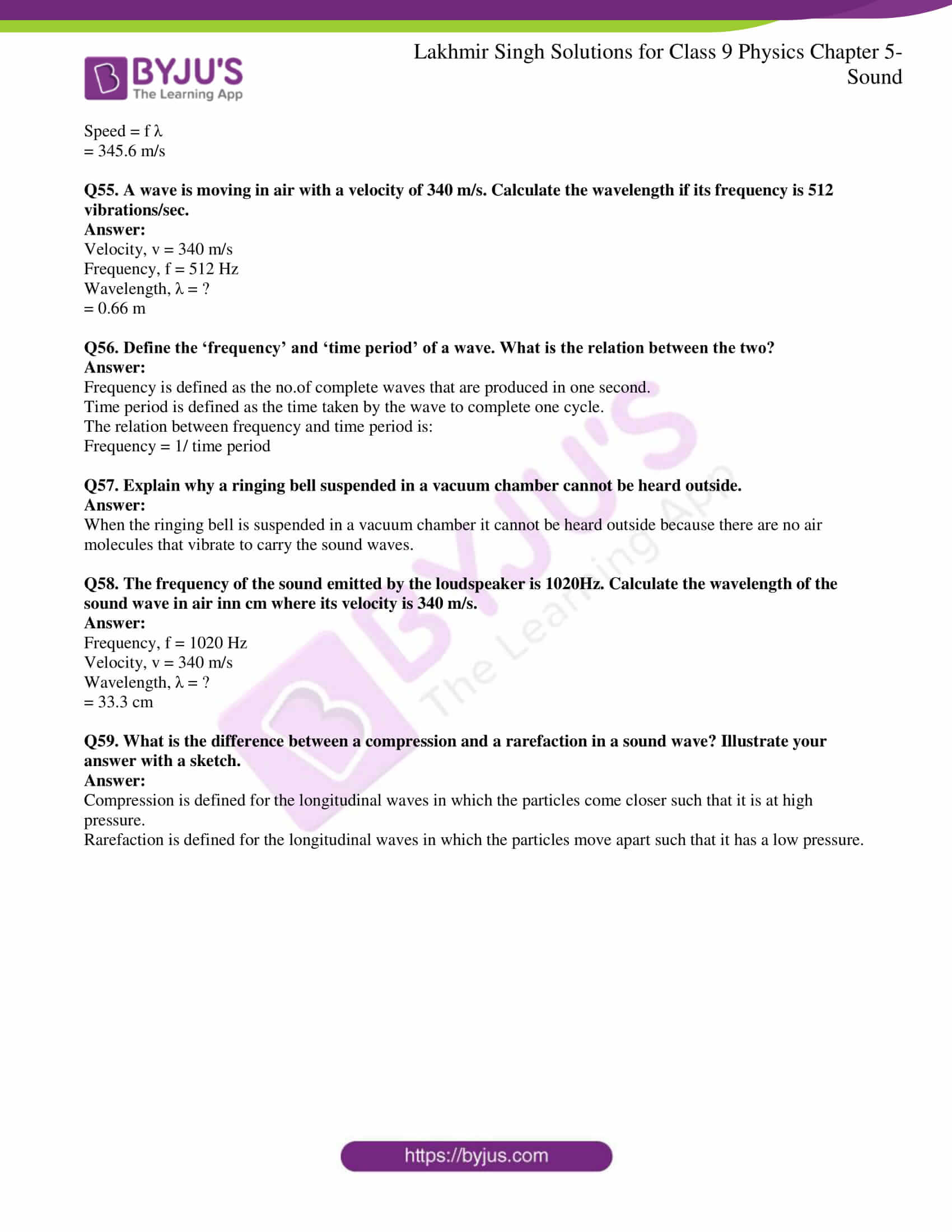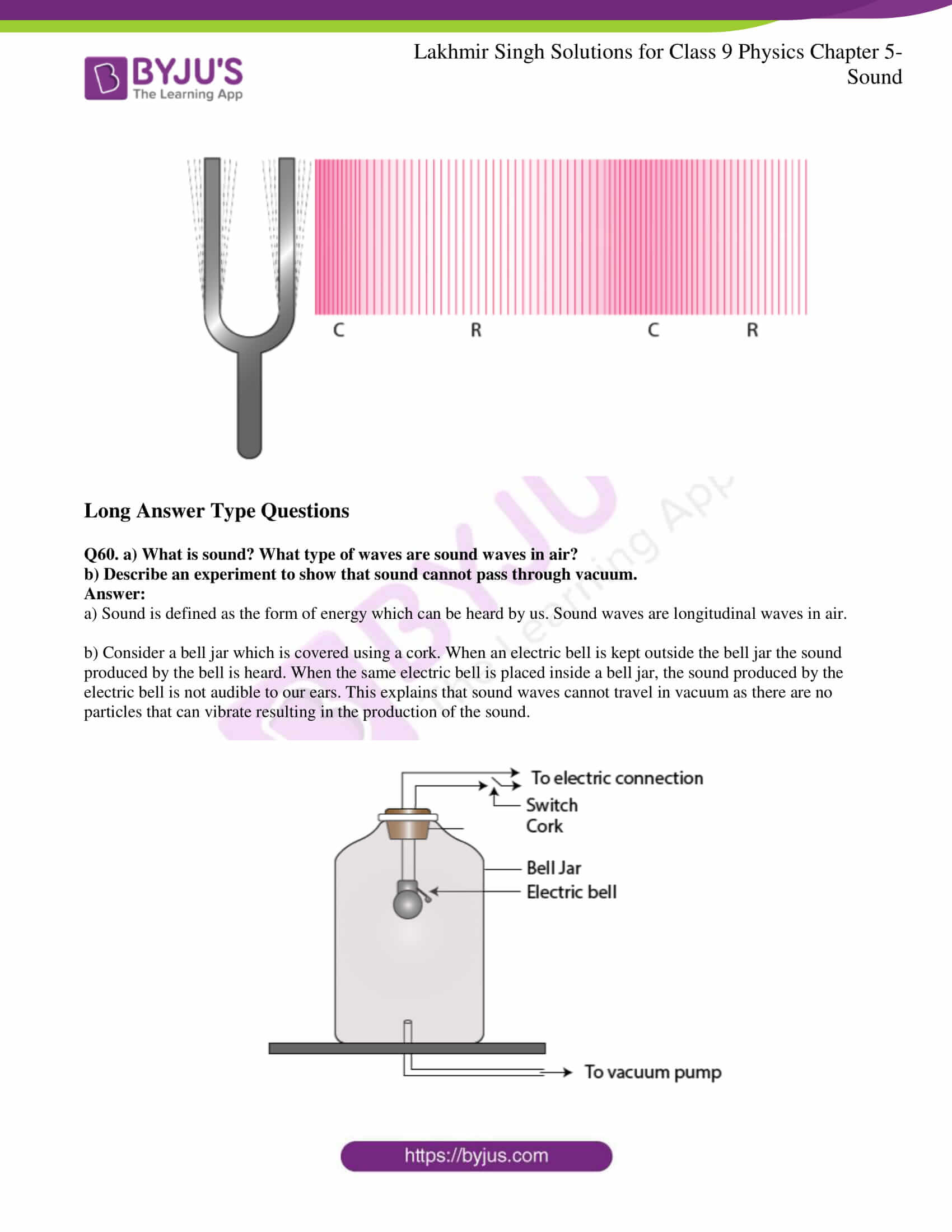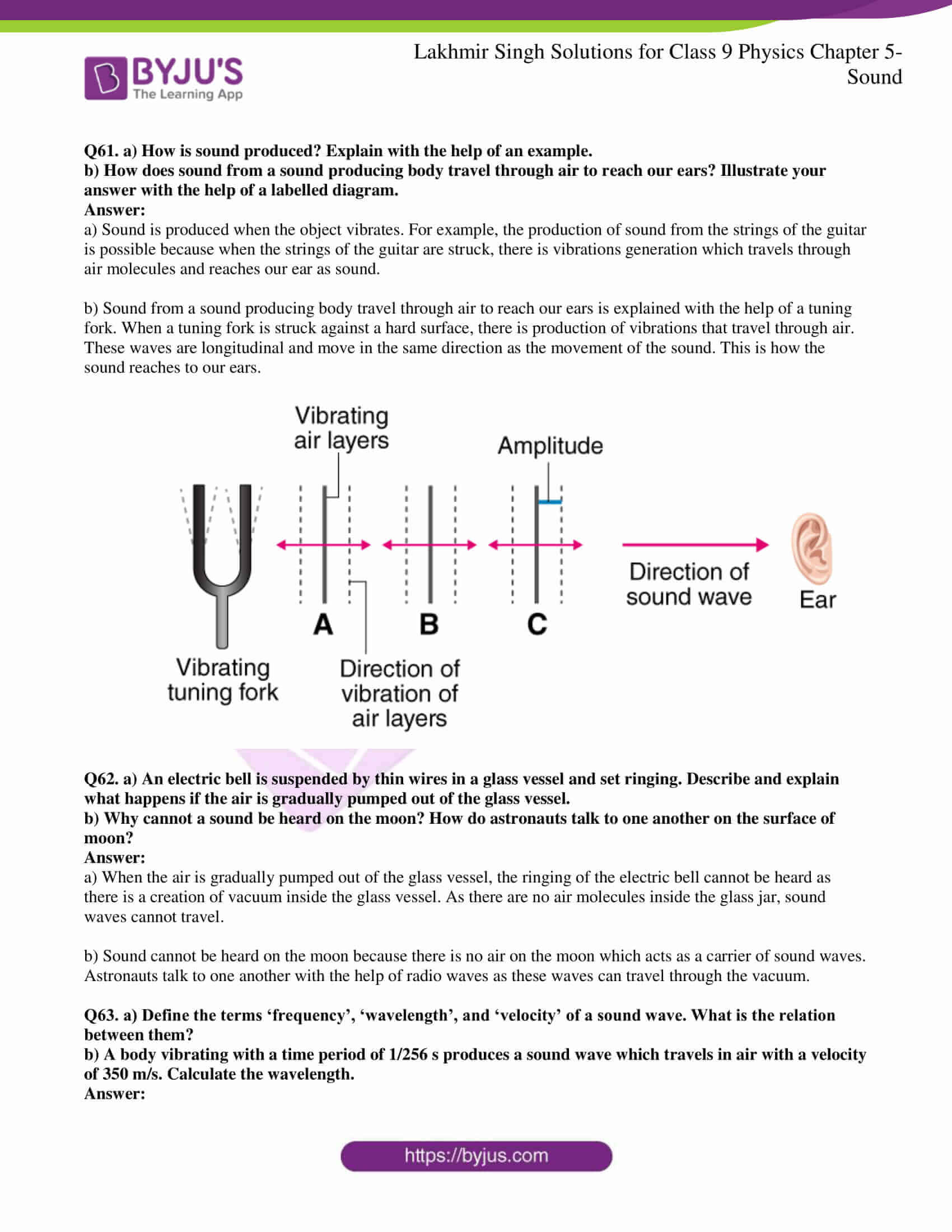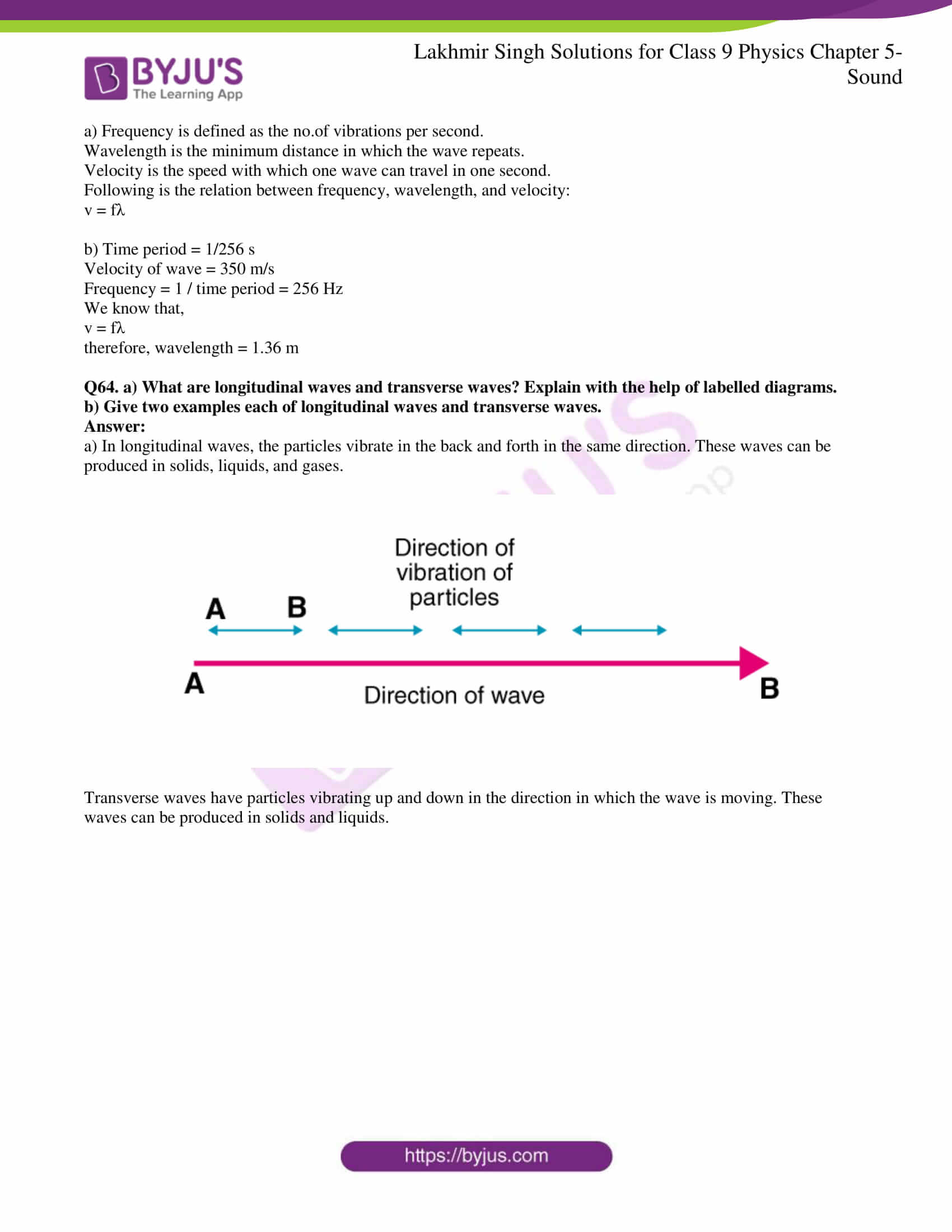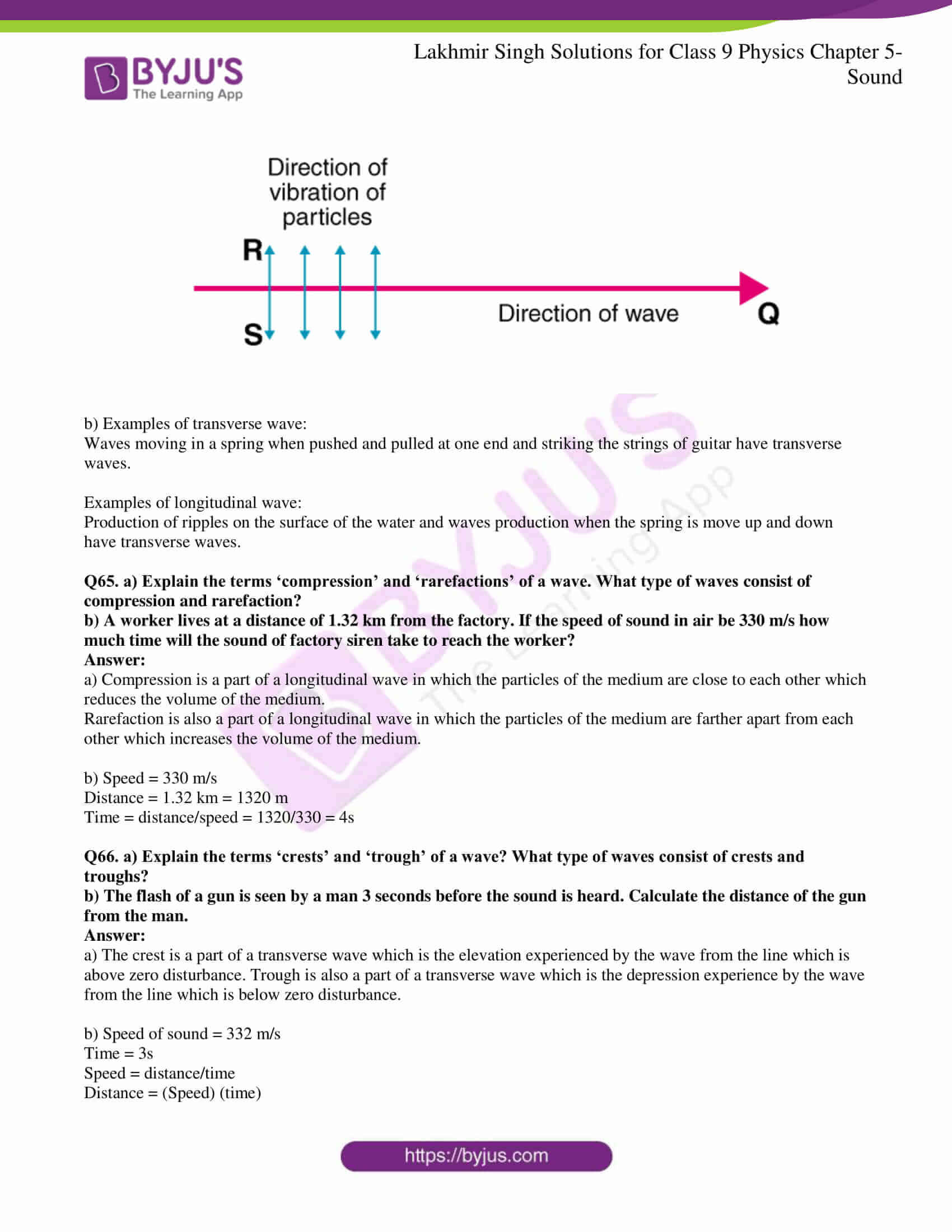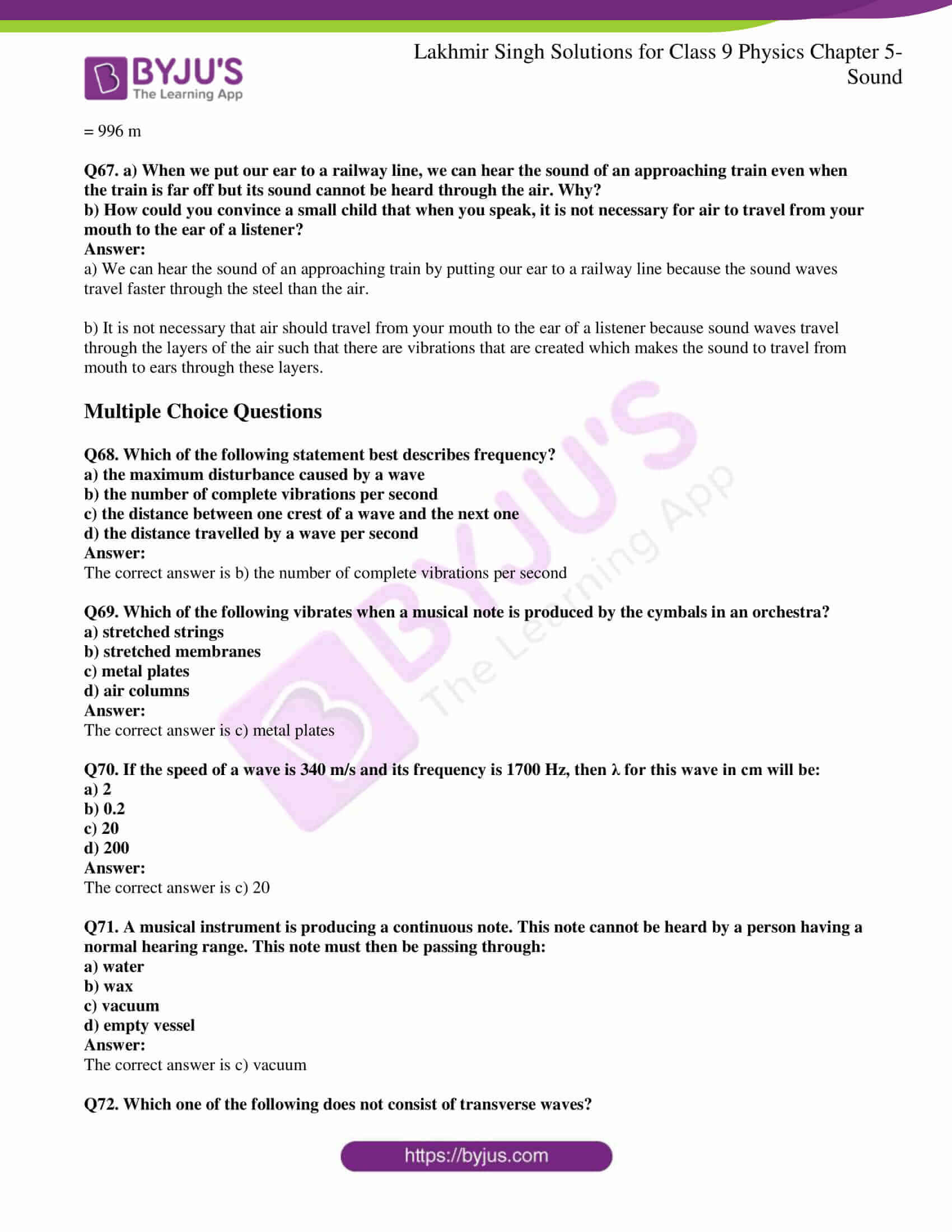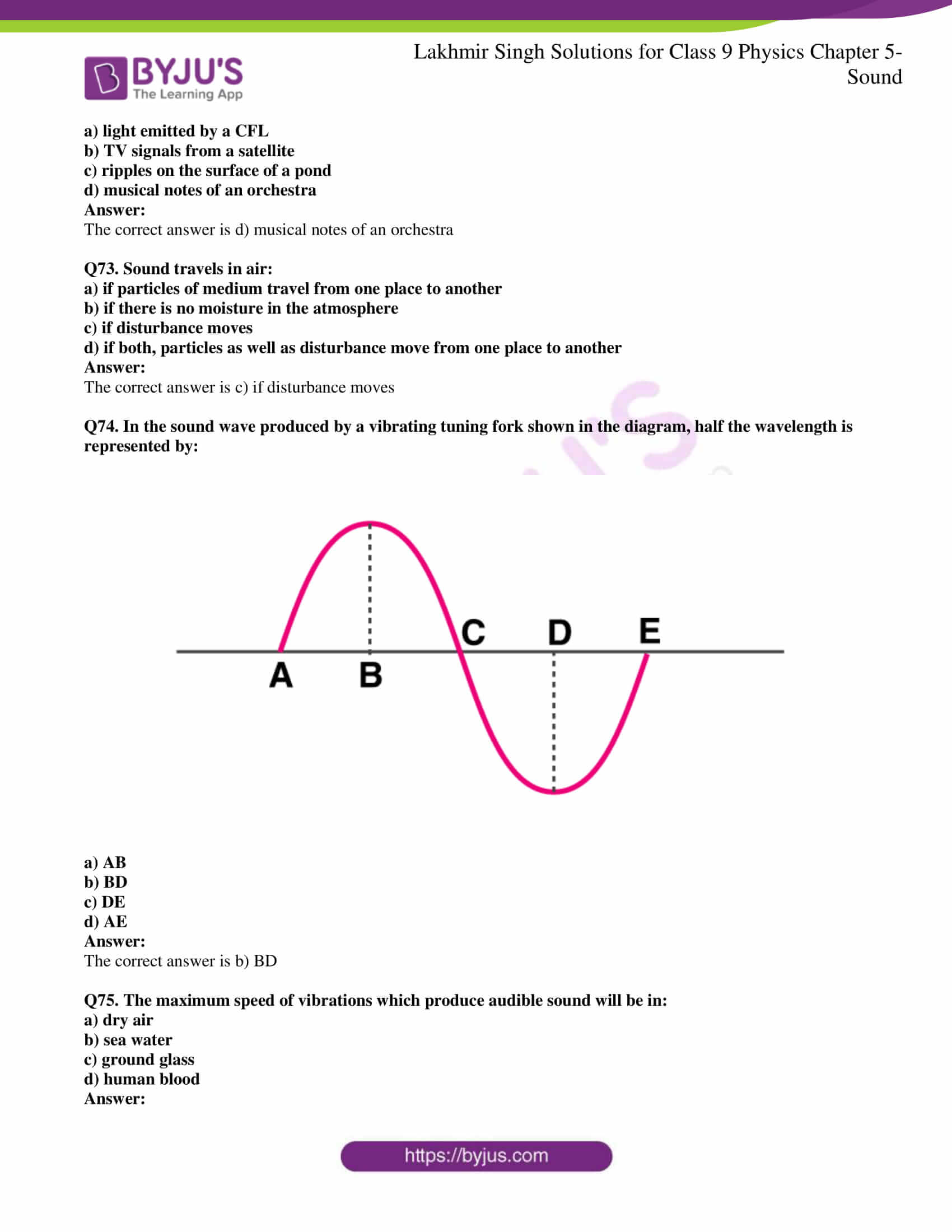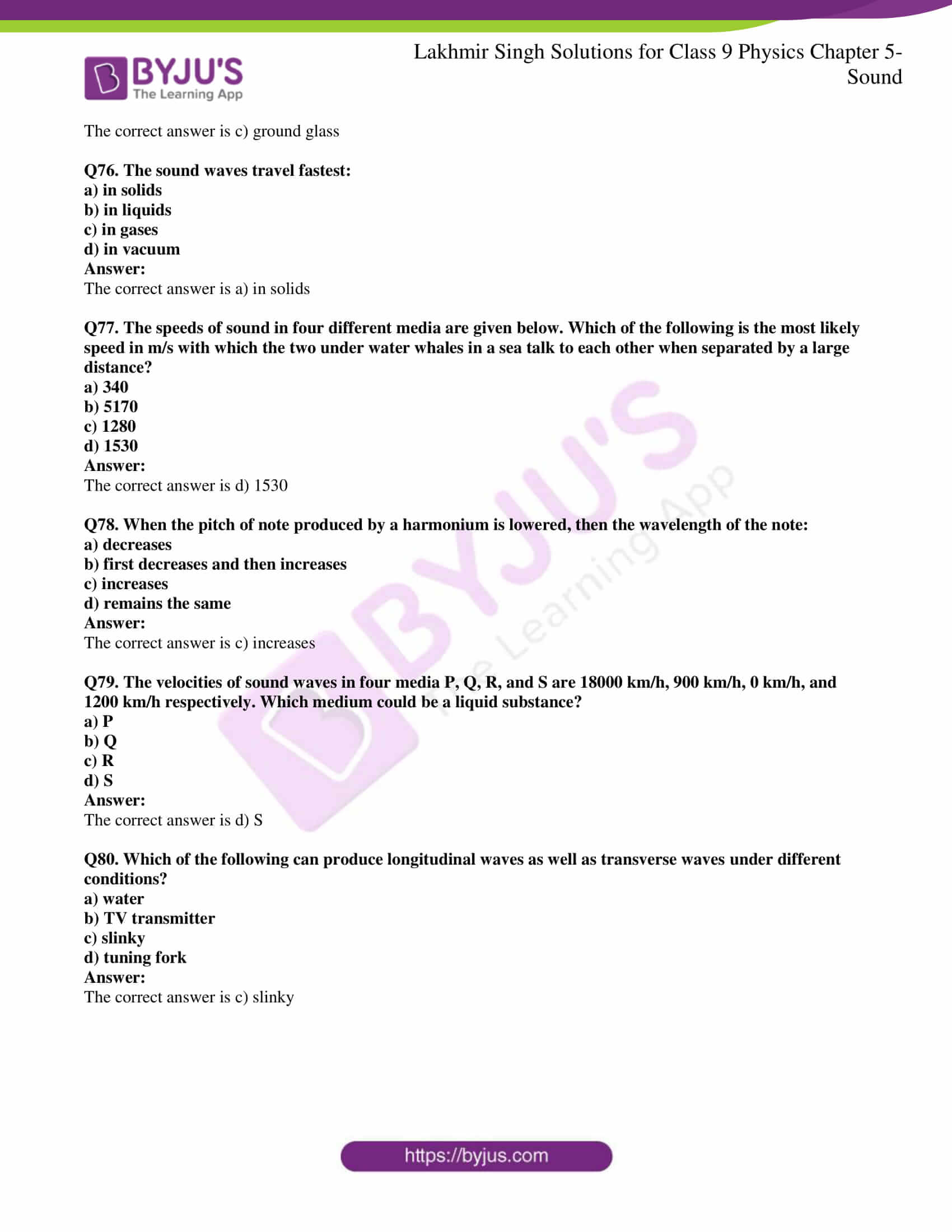Page No: 185

### Very Short Answer Type Questions

Q1. Can sound travel through

a) iron

b) water?

Sound can travel through both iron as well as water.

Q2. Can sound travel through vacuum?

No, sound cannot travel through vacuum.

Q3. Name the type of waves which are used by astronauts to communicate with one another on moon.

Astronauts use radio waves for communications.

Q4. Name one solid, one liquid, and one gas through which sound can travel.

Solid: table

Liquid: water

Gas: air

Q5. Which of the following cannot transmit sound?

Water, vacuum, aluminium, oxygen gas

Vacuum

Q6. Name the physical quantity whose SI unit is ‘hertz’.

Frequency

Q7. What is the SI unit of frequency?

Hertz is the SI unit of frequency.

Q8. What type of wave is represented:

a) by density-distance graph?

b) by displacement-distance graph?

a) Longitudinal wave

b) Transverse wave

Q9. Is the speed of sound more in water or in steel?

Speed of sound is more in steel than in water.

Q10. In which medium sound travels faster: air or iron?

Sound travels faster in iron than in air.

Q11. In which medium sound travels fastest: air, water, or steel?

Sound travels fastest in steel.

Q12. Out of solids, liquids, and gases:

a) in which medium sound travels slowest?

b) in which medium sound travels fastest?

a) Sound travels slowest in gases

b) Sound travels fastest in solids

Q13. Which of the following is the speed of sound in copper and which in aluminum?

a) 5100 m/s

b) 1500 m/s

c) 3750 m/s

a) Speed of sound in copper is 3750 m/s

b) Speed of sound in aluminum is 5100 m/s

Q14. If you want to hear a train approaching from far away, why is it more convenient to put the ear to the track?

It is more convenient to put the ear to the track because sound waves travel faster in solids than in air.

Q15. What is the speed of sound:

a) in air

b) in water

c) in iron

a) Air is 344 m/s

b) Water is 1498 m/s

c) Iron is 5130 m/s

Q16. What name is given to those aircrafts which fly at speeds greater than the speed of sound?

Supersonic aircrafts

Q17. A jet aircraft flies at a speed of 410 m/s. What is this speed known as?

Supersonic speed

Q18. What is meant by supersonic speed?

Supersonic speed means the speed of an object which greater than the speed of sound.

Q19. State one observation from everyday life which shows that sound travels much more slow than light.

One observation from everyday life which shows that sound travels much more slow than light is during monsoons. We first see the lightning and then hear the sound of thunder.

Q20. Name the two types of waves which can be generated in a long flexible spring.

The two types of waves are longitudinal waves and transverse waves.

Q21. A stone is dropped on the surface of water in a pond. Name the type of waves produced.

Transverse waves.

Q22. Name the type of waves produced when a tuning fork is truck in air.

Longitudinal waves

Q23. What is the general name of the waves consisting of:

a) compressions and rarefactions

b) crests and troughs

a) Longitudinal waves

b) Transverse waves

Q24. State the general name of the waves in which the particles of the medium vibrate:

a) in the same direction as wave

b) at right angles to the direction of wave

a) Longitudinal waves

b) Transverse waves

Q25. What type of waves are illustrated by the movement of a rope whose one end is fixed to a pole and the other end is moved up and down?

Transverse waves

Q26. What should an object do to produce sound?

An object produces sound there is a creation of vibrations.

Q27. What is the name of the strings which vibrate in our voice box when we talk?

Vocal cords in our voice box produces sound.

Q28. Name the device which is used to produce sound in laboratory experiments.

Tuning fork is the device which is used for producing sound in laboratory experiments.

Q29. What is the nature of sound waves in air?

The nature of sound waves in air is longitudinal waves.

Q30. What conclusion can be obtained from the observation that when the prongs of a sound making tuning fork touch the surface of water in a beaker, the water gets splashed?

The conclusion that can be obtained from the observation when the prongs of tuning fork touches the surface of water is that the vibrations from the tuning forks are transmitted to the surrounding medium.

Q31. State whether the following statement is true or false:

Sound produced by a vibrating body travels to our ears by the actual movement of air.

False

Q32. Which of the following travels slowest in air and which one fastest

Supersonic aircraft, light, sound

Slowest is the sound

Fastest is the light

Q33. Which term is used to denote a speed greater than the speed of sound?

Supersonic is the terms that is used for denoting the speed which is greater than the speed of sound.

Q34. In which medium sound travels faster: air or hydrogen?

Sound travels faster in hydrogen.

Q35. A tuning fork has a number 256 marked on it. What does this number signify?

The number 256 on the tuning fork signifies the frequency of the tuning fork.

Q36. What is the time-period of a tuning fork whose frequency is 200 Hz?

Frequency = 200 Hz

Frequency = 1/ time period

Time period = 1/ frequency

= 5 × 10-9 s

Q37. Calculate the frequency of a wave whose time-period is 0.02s.

Frequency = 1/ time period

= 1/0.02

= 50 Hz

Q38. What will be the change in the wavelength of a sound wave in air if its frequency is doubled?

Velocity of sound is the product of frequency and wavelength. The speed of sound in air is constant. Therefore, when the frequency is doubled, wavelength reduces to half.

Q39. If 20 waves are produced per second, what is the frequency in hertz?

The frequency in hertz is 20 hertz as the hertz is the representation of number of waves produced per second.

Q40. Fill in the blanks with suitable words:

a) Sound is caused by ………..

b) A sound wave consists of places of higher pressure called ………. and places of ……….. pressure called ………..

c) Wave speed in meters per second equals frequency in ……… multiplied by ……….. in ………..

d) Sound cannot travel through …………

e) The speed of sound in a solid is ……… than the speed of sound in air.

f) When the frequency of the sound is increased, the wavelength ……..

a) Vibrations

b) Compression, lower, and rarefaction

c) Hertz, wavelength, and meters

d) Vacuum

e) Greater

f) Decreases

Q41. What is vacuum? Explain why sound cannot travel through vacuum?

Vacuum is defined as the empty space in which there are no matter particles present. Sound cannot travel through vacuum as there is no particles present for vibrations to take place.

Q42. Explain the term ‘amplitude’ of a wave. Draw the diagram of a wave and mark its amplitude on it.

Amplitude is defined as the maximum displacement of the particle from its original undisturbed position.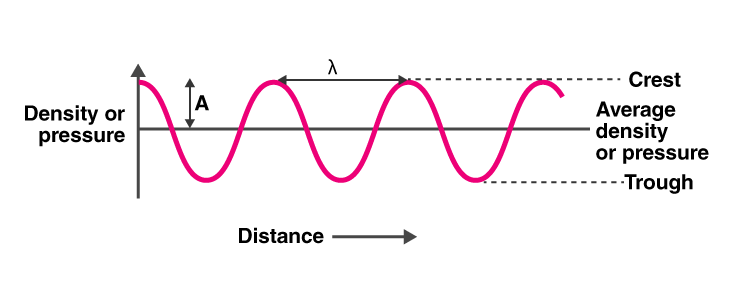Q43. a) Distinguish between longitudinal and transverse waves.

b) Are sound waves longitudinal or transverse?

a) In longitudinal waves, the particles move back and forth in the same direction whereas in transverse waves, the particles move up and down.

Longitudinal waves can be produced in solids, liquids, and in gases whereas transverse waves are produced only in solids and liquids.

b) Sound is a longitudinal wave.

Q44. A cricket ball is seen to hit the bat first and the sound of hitting is heard a little later. Why?

We see the bat hitting the ball first and then hear the sound because light travels faster than the sound.

Q45. Explain why the flash of lightning reaches us first and the sound of thunder is heard a little later.

The flash of lightning reaches us first and then the sound of thunder because light waves travel faster than the sound waves.

Q46. Explain why the flash of a gun shot reaches us before the sound of the gun shot.

The flash of gun shot reaches first because the light waves travel faster than the sound waves of the gun shot.

Q47. Which of the following terms apply to sound waves in air and which to water waves?

Transverse, rarefaction, trough, crest, compression, longitudinal

Sound waves in air: longitudinal, compression, rarefaction

Water waves: transverse, crest, trough

Q48. a) Name four ways in which sound can be produced.

b) Calculate the speed of a sound wave whose frequency is 2 kHz and wavelength 65 cm.

a) Following are the ways in which sound can be produced:

i) by vibrating the strings

ii) by vibrating membranes

iii) by vibrating the plates

b) Speed of sound wave = (frequency)(wavelength)

Frequency, f = 2 kHz

Wavelength, λ = 65 cm = 0.65 m

Therefore, speed = 1300 m/s

Q49. If a ringing bicycle bell is held tightly by hand, it stops producing sound. Why?

The ringing bicycle bell does not produce sound when it is held tightly with hand because the vibrations are stopped and so the sound production stops.

Q50. Which object is vibrating when the following sounds are produced?

a) the sound of a sitar

b) the sound of a table

c) the sound of a tuning fork

d) the buzzing of a bee or mosquito

e) the sound of a flute

a) Vibrations in the stretched strings of sitar

b) Vibrations from the stretched membrane

c) Vibrations from the prongs

d) Vibrations from the wings

e) Vibrations from the air columns

Q51. Give reason for the following:

In most of the cases, we cannot see the vibrations of a sound producing objects with our eyes.

We cannot see the vibrations because the time interval between the two successive vibrations is very less that it hard to observe them.

Q52. Describe a simple experiment to show that the prongs of a sound producing tuning fork are vibrating.

Fill the beaker with water till its brim. Now touch the surface of the water with the prongs of the vibrating tuning forks. It can be seen that water starts to splash. This shows that the tuning fork is vibrating.Q53. When we open a gas tap for a few seconds, the sound of escaping gas is heard first but the smell of gas comes later. Why?

The sound is heard before because the sound waves travel through the air molecules by creating vibrations while the smell reaches to us through the movement of the air molecules.

Q54. A sound signal of 128 vibrations per second has a wavelength of 2.7m. Calculate the speed with which the wave travels.

Frequency is the no.of vibrations produced per second = 128 Hz

Wavelength, λ = 2.7 m

Speed = f λ

= 345.6 m/s

Q55. A wave is moving in air with a velocity of 340 m/s. Calculate the wavelength if its frequency is 512 vibrations/sec.

Velocity, v = 340 m/s

Frequency, f = 512 Hz

Wavelength, λ = ?

= 0.66 m

Q56. Define the ‘frequency’ and ‘time period’ of a wave. What is the relation between the two?

Frequency is defined as the no.of complete waves that are produced in one second.

Time period is defined as the time taken by the wave to complete one cycle.

The relation between frequency and time period is:

Frequency = 1/ time period

Q57. Explain why a ringing bell suspended in a vacuum chamber cannot be heard outside.

When the ringing bell is suspended in a vacuum chamber it cannot be heard outside because there are no air molecules that vibrate to carry the sound waves.

Q58. The frequency of the sound emitted by the loudspeaker is 1020Hz. Calculate the wavelength of the sound wave in air inn cm where its velocity is 340 m/s.

Frequency, f = 1020 Hz

Velocity, v = 340 m/s

Wavelength, λ = ?

= 33.3 cm

Q59. What is the difference between a compression and a rarefaction in a sound wave? Illustrate your answer with a sketch.

Compression is defined for the longitudinal waves in which the particles come closer such that it is at high pressure.

Rarefaction is defined for the longitudinal waves in which the particles move apart such that it has a low pressure.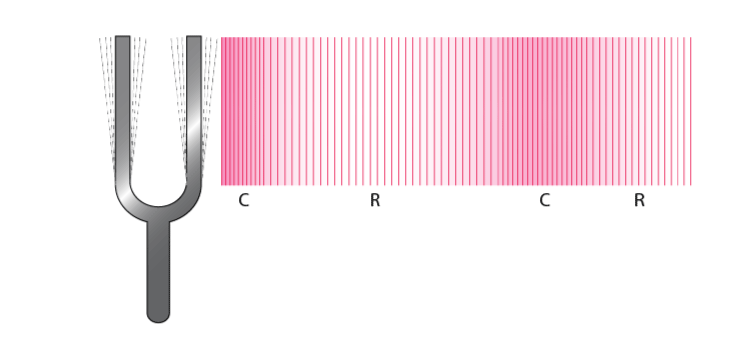Q60. a) What is sound? What type of waves are sound waves in air?

b) Describe an experiment to show that sound cannot pass through vacuum.

a) Sound is defined as the form of energy which can be heard by us. Sound waves are longitudinal waves in air.

b) Consider a bell jar which is covered using a cork. When an electric bell is kept outside the bell jar the sound produced by the bell is heard. When the same electric bell is placed inside a bell jar, the sound produced by the electric bell is not audible to our ears. This explains that sound waves cannot travel in vacuum as there are no particles that can vibrate resulting in the production of the sound.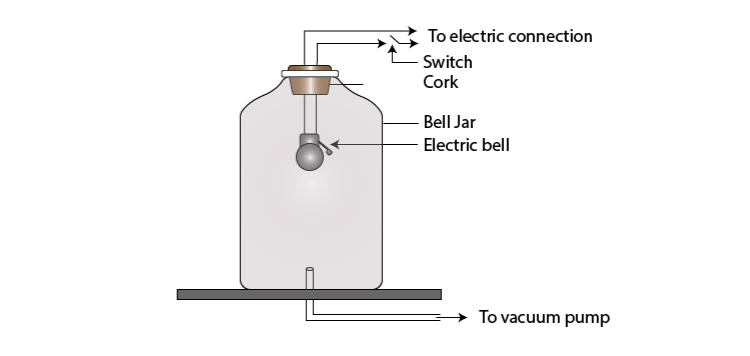Q61. a) How is sound produced? Explain with the help of an example.

b) How does sound from a sound producing body travel through air to reach our ears? Illustrate your answer with the help of a labelled diagram.

a) Sound is produced when the object vibrates. For example, the production of sound from the strings of the guitar is possible because when the strings of the guitar are struck, there is vibrations generation which travels through air molecules and reaches our ear as sound.

b) Sound from a sound producing body travel through air to reach our ears is explained with the help of a tuning fork. When a tuning fork is struck against a hard surface, there is production of vibrations that travel through air. These waves are longitudinal and move in the same direction as the movement of the sound. This is how the sound reaches to our ears.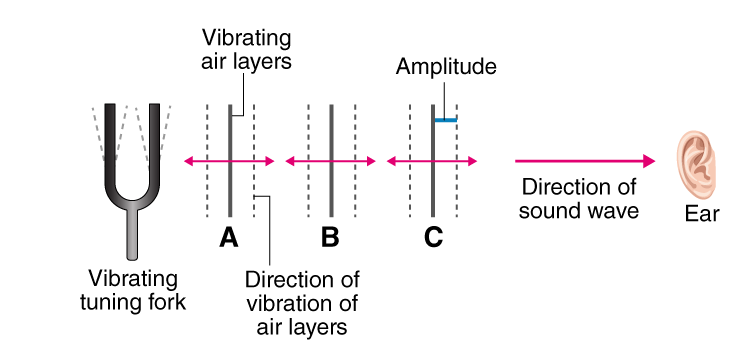Q62. a) An electric bell is suspended by thin wires in a glass vessel and set ringing. Describe and explain what happens if the air is gradually pumped out of the glass vessel.

b) Why cannot a sound be heard on the moon? How do astronauts talk to one another on the surface of moon?

a) When the air is gradually pumped out of the glass vessel, the ringing of the electric bell cannot be heard as there is a creation of vacuum inside the glass vessel. As there are no air molecules inside the glass jar, sound waves cannot travel.

b) Sound cannot be heard on the moon because there is no air on the moon which acts as a carrier of sound waves. Astronauts talk to one another with the help of radio waves as these waves can travel through the vacuum.

Q63. a) Define the terms ‘frequency’, ‘wavelength’, and ‘velocity’ of a sound wave. What is the relation between them?

b) A body vibrating with a time period of 1/256 s produces a sound wave which travels in air with a velocity of 350 m/s. Calculate the wavelength.

a) Frequency is defined as the no.of vibrations per second.

Wavelength is the minimum distance in which the wave repeats.

Velocity is the speed with which one wave can travel in one second.

Following is the relation between frequency, wavelength, and velocity:

v = fλ

b) Time period = 1/256 s

Velocity of wave = 350 m/s

Frequency = 1 / time period = 256 Hz

We know that,

v = fλ

therefore, wavelength = 1.36 m

Q64. a) What are longitudinal waves and transverse waves? Explain with the help of labelled diagrams.

b) Give two examples each of longitudinal waves and transverse waves.

a) In longitudinal waves, the particles vibrate in the back and forth in the same direction. These waves can be produced in solids, liquids, and gases.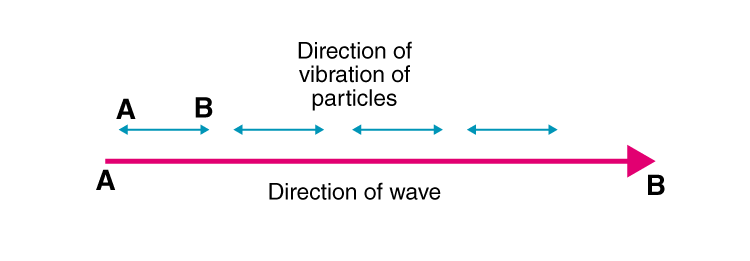Transverse waves have particles vibrating up and down in the direction in which the wave is moving. These waves can be produced in solids and liquids.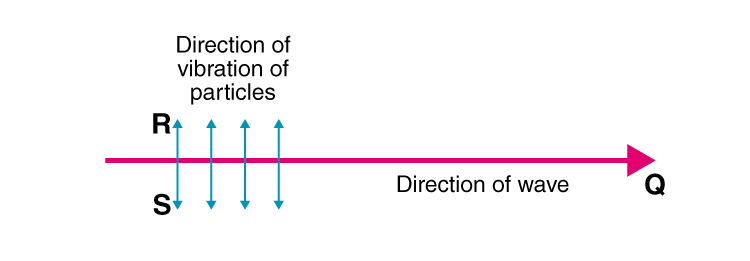b) Examples of transverse wave:

Waves moving in a spring when pushed and pulled at one end and striking the strings of guitar have transverse waves.

Examples of longitudinal wave:

Production of ripples on the surface of the water and waves production when the spring is move up and down have transverse waves.

Q65. a) Explain the terms ‘compression’ and ‘rarefactions’ of a wave. What type of waves consist of compression and rarefaction?

b) A worker lives at a distance of 1.32 km from the factory. If the speed of sound in air be 330 m/s how much time will the sound of factory siren take to reach the worker?

a) Compression is a part of a longitudinal wave in which the particles of the medium are close to each other which reduces the volume of the medium.

Rarefaction is also a part of a longitudinal wave in which the particles of the medium are farther apart from each other which increases the volume of the medium.

b) Speed = 330 m/s

Distance = 1.32 km = 1320 m

Time = distance/speed = 1320/330 = 4s

Q66. a) Explain the terms ‘crests’ and ‘trough’ of a wave? What type of waves consist of crests and troughs?

b) The flash of a gun is seen by a man 3 seconds before the sound is heard. Calculate the distance of the gun from the man.

a) The crest is a part of a transverse wave which is the elevation experienced by the wave from the line which is above zero disturbance. Trough is also a part of a transverse wave which is the depression experience by the wave from the line which is below zero disturbance.

b) Speed of sound = 332 m/s

Time = 3s

Speed = distance/time

Distance = (Speed) (time)

= 996 m

Q67. a) When we put our ear to a railway line, we can hear the sound of an approaching train even when the train is far off but its sound cannot be heard through the air. Why?

b) How could you convince a small child that when you speak, it is not necessary for air to travel from your mouth to the ear of a listener?

a) We can hear the sound of an approaching train by putting our ear to a railway line because the sound waves travel faster through the steel than the air.

b) It is not necessary that air should travel from your mouth to the ear of a listener because sound waves travel through the layers of the air such that there are vibrations that are created which makes the sound to travel from mouth to ears through these layers.

### Multiple Choice Questions

Q68. Which of the following statement best describes frequency?

a) the maximum disturbance caused by a wave

b) the number of complete vibrations per second

c) the distance between one crest of a wave and the next one

d) the distance travelled by a wave per second

The correct answer is b) the number of complete vibrations per second

Q69. Which of the following vibrates when a musical note is produced by the cymbals in an orchestra?

a) stretched strings

b) stretched membranes

c) metal plates

d) air columns

The correct answer is c) metal plates

Q70. If the speed of a wave is 340 m/s and its frequency is 1700 Hz, then λ for this wave in cm will be:

a) 2

b) 0.2

c) 20

d) 200

The correct answer is c) 20

Q71. A musical instrument is producing a continuous note. This note cannot be heard by a person having a normal hearing range. This note must then be passing through:

a) water

b) wax

c) vacuum

d) empty vessel

The correct answer is c) vacuum

Q72. Which one of the following does not consist of transverse waves?

a) light emitted by a CFL

b) TV signals from a satellite

c) ripples on the surface of a pond

d) musical notes of an orchestra

The correct answer is d) musical notes of an orchestra

Q73. Sound travels in air:

a) if particles of medium travel from one place to another

b) if there is no moisture in the atmosphere

c) if disturbance moves

d) if both, particles as well as disturbance move from one place to another

The correct answer is c) if disturbance moves

Q74. In the sound wave produced by a vibrating tuning fork shown in the diagram, half the wavelength is represented by: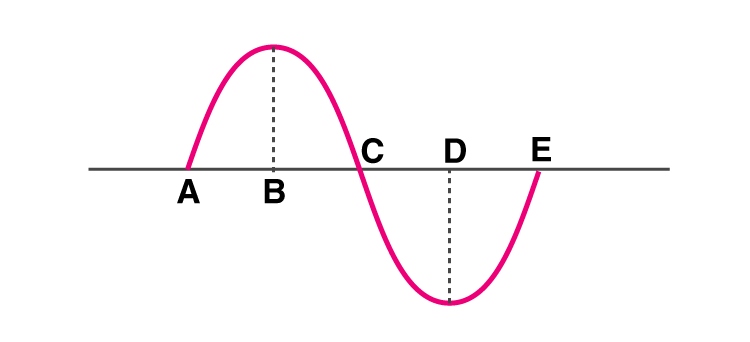a) AB

b) BD

c) DE

d) AE

The correct answer is b) BD

Q75. The maximum speed of vibrations which produce audible sound will be in:

a) dry air

b) sea water

c) ground glass

d) human blood

The correct answer is c) ground glass

Q76. The sound waves travel fastest:

a) in solids

b) in liquids

c) in gases

d) in vacuum

The correct answer is a) in solids

Q77. The speeds of sound in four different media are given below. Which of the following is the most likely speed in m/s with which the two under water whales in a sea talk to each other when separated by a large distance?

a) 340

b) 5170

c) 1280

d) 1530

The correct answer is d) 1530

Q78. When the pitch of note produced by a harmonium is lowered, then the wavelength of the note:

a) decreases

b) first decreases and then increases

c) increases

d) remains the same

The correct answer is c) increases

Q79. The velocities of sound waves in four media P, Q, R, and S are 18000 km/h, 900 km/h, 0 km/h, and 1200 km/h respectively. Which medium could be a liquid substance?

a) P

b) Q

c) R

d) S

The correct answer is d) S

Q80. Which of the following can produce longitudinal waves as well as transverse waves under different conditions?

a) water

b) TV transmitter

d) tuning fork